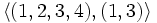# Subgroup structure of symmetric group:S4

View subgroup structure of particular groups | View other specific information about symmetric group:S4

The symmetric group of degree four has many subgroups.

Note that since$S_4$ is a complete group, every automorphism is inner, so the classification of subgroups upto conjugacy is equivalent to the classification of subgroups upto automorphism. In other words, every subgroup is an automorph-conjugate subgroup.

1. The trivial subgroup. Isomorphic to trivial group.(1)
2. S2 in S4: The two-element subgroup generated by a transposition, such as$(1,2)$. Isomorphic to cyclic group of order two. (6)
3. Subgroup generated by double transposition in S4: The two-element subgroup generated by a double transposition, such as$(1,2)(3,4)$. Isomorphic to cyclic group of order two. (3)
4. Non-normal Klein four-subgroups of symmetric group:S4The four-element subgroup generated by two disjoint transpositions, such as$\langle (1,2) \ , \ (3,4) \rangle$. Isomorphic to Klein four-group. (3)
5. Normal Klein four-subgroup of symmetric group:S4: The unique four-element subgroup comprising the identity and the three double transpositions. Isomorphic to Klein four-group. (1)
6. Z4 in S4: The four-element subgroup spanned by a 4-cycle. Isomorphic to cyclic group of order four.(3)
7. D8 in S4: The eight-element subgroup spanned by a 4-cycle and a transposition that conjugates this cycle to its inverse. Isomorphic to dihedral group of order eight. This is also a 2-Sylow subgroup. (3)
8. A3 in S4: The three-element subgroup spanned by a three-cycle. Isomorphic to cyclic group of order three.(4)
9. S3 in S4: The six-element subgroup comprising all permutations that fix one element. Isomorphic to symmetric group on three elements. (4)
10. A4 in S4: The alternating group: the subgroup of all even permutations. Isomorphic to alternating group:A4.(1)
11. The whole group.(1)

## Tables for quick information

### Table classifying subgroups up to automorphisms

Automorphism class of subgroups Representative Isomorphism class Number of conjugacy classes Size of each conjugacy class Isomorphism class of quotient (if exists) Subnormal depth (if subnormal)
trivial subgroup$\{ () \}$ trivial group 1 1 symmetric group:S4 1
S2 in S4$\{ (), (1,2) \}$ cyclic group:Z2 1 6 -- --
subgroup generated by double transposition in S4$\{ (), (1,2)(3,4) \}$ cyclic group:Z2 1 3 -- 2
A3 in S4$\{ (), (1,2,3), (1,3,2) \}$ cyclic group:Z3 1 4 -- --
Z4 in S4$\langle (1,2,3,4) \rangle$ cyclic group:Z4 1 3 -- --
normal Klein four-subgroup of S4$\{ (), (1,2)(3,4), (1,3)(2,4), (1,4)(2,3) \rangle$ Klein four-group 1 1 symmetric group:S3 1
non-normal Klein four-subgroups of S4$\langle (1,2), (3,4) \rangle$ Klein four-group 1 3 -- --
S3 in S4$\langle (1,2,3), (1,2) \rangle$ symmetric group:S3 1 4 -- --
D8 in S4$\langle (1,2,3,4), (1,3) \rangle$ dihedral group:D8 1 3 -- --
A4 in S4$\langle (1,2,3), (1,2)(3,4) \rangle$ alternating group:A4 1 1 cyclic group:Z2 1
whole group$\langle (1,2,3,4), (1,2) \rangle$ symmetric group:S4 1 1 trivial group 0

### Table classifying isomorphism types of subgroups

Group name Order Second part of GAP ID (first part is order) Occurrences as subgroup Conjugacy classes of occurrence as subgroup Occurrences as normal subgroup Occurrences as characteristic subgroup
Trivial group 1 1 1 1 1 1
Cyclic group:Z2 2 1 9 2 0 0
Cyclic group:Z3 3 1 4 1 0 0
Cyclic group:Z4 4 1 3 1 0 0
Klein four-group 4 2 4 2 1 1
Symmetric group:S3 6 1 4 1 0 0
Dihedral group:D8 8 3 3 1 0 0
Alternating group:A4 12 3 1 1 1 1
Symmetric group:S4 24 12 1 1 1 1
Total -- -- 30 11 4 4

### Table listing number of subgroups by order

Note that these orders satisfy the congruence condition on number of subgroups of given prime power order: the number of subgroups of order$p^r$ is congruent to$1$ modulo$p$.

Group order Occurrences as subgroup Conjugacy classes of occurrence as subgroup Occurrences as normal subgroup Occurrences as characteristic subgroup
1 1 1 1 1
2 9 2 0 0
3 4 1 0 0
4 7 3 1 1
6 4 1 0 0
8 3 1 0 0
12 1 1 1 1
24 1 1 1 1
Total 30 11 4 4

### Table listing numbers of subgroups by group property

Group property Occurrences as subgroup Conjugacy classes of occurrence as subgroup Occurrences as normal subgroup Occurrences as characteristic subgroup
Cyclic group 17 5 1 1
Abelian group 21 7 2 2
Nilpotent group 24 8 2 2
Solvable group 30 11 5 5

### Table listing numbers of subgroups by subgroup property

Subgroup property Occurences as subgroup Conjugacy classes of occurrences as subgroup Automorphism classes of occurrences as subgroup
Subgroup 30 11 11
Normal subgroup 5 5 5
Characteristic subgroup 5 5 5